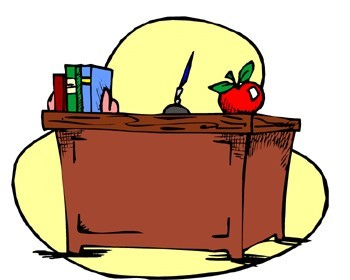Like   Tweet   +1
 /* styles */

## June 2017

 table div table+table+table+table div table{width:100%;padding:0}table div table+table+table+table div table img{width:96.23%;padding:0;float:none}table div table+table+table+table div table td{width:100%;padding:0 1.88% 18px}/* styles */## Edupaths Content CreationThe EduPaths Team is looking for educators who would be strong contributors of content in the areas of Assessment, Data or Leadership. Their second summer of EduPaths Content Creation will be starting soon.

 table div table+table+table+table+table+table+table div table{width:100%;padding:0}table div table+table+table+table+table+table+table div table img{width:96.23%;padding:0;float:none}table div table+table+table+table+table+table+table div table td{width:100%;padding:0 1.88% 18px}/* styles */## Summer Resources for StudentsThe MeL team would like to remind everyone of the great resources Michigan residents have free access to through the Michigan eLibrary. Families can find resources to help ease the summer slide, read eBooks, conduct research, create reading lists, and prepare for college, careers, and high stakes tests like the SATⓇ. This year's theme is "Build a Better World"

 table div table+table+table+table+table+table+table+table+table+table div table{width:100%;padding:0}table div table+table+table+table+table+table+table+table+table+table div table img{width:96.23%;padding:0;float:none}table div table+table+table+table+table+table+table+table+table+table div table td{width:100%;padding:0 1.88% 18px}/* styles */## REMINDER...Summer WorkshopsIt is not too late to sign up for our five upcoming workshops this Summer!
Registration is available on Wisdomwhere.
Wisdomwhere CCISD
Wisdomwhere GOISD
(Peer to CCISD to find and register)

_______________________________________

Learn the Whole Caboodle on Moodle
3 SCECHs
June 23 GOISD
June 30 CCISD
12:30p.m.-4:00 p.m.
Join REMC 1's Moodle Evangelists, Steve Kass and Mark Nordin, for the latest updates and features to Moodle, how it can be used in your classroom, and tips on designing your own courses in this robust learning management system. Time will also be allotted to work on your course under the guidance of seasoned Moodle users!
______________________________________

G Suite for Education
5 SCECHs
July 20 CCISD
August 10 GOISD
9:00 a.m.-3:00 p.m.
Google recently overhauled their Apps for Education and with the new changes, comes a new name--G Suite. Steve Kass, REMC1's Google Certified Trainer, will walk you through some of these changes and will help assist you with implementing the new G Suite into your classroom!
_____________________________________

Classroom Maker
5 SCECHs
July 28 CCISD
August 18 GOISD
9:00 a.m.-3:00 p.m.
The maker movement is a broad term that integrates "play" with learning as students design, create, innovate, and "tinker" with tools. This workshop will prepare teachers to utilize the makerspace kit that is available for teachers to check-out from REMC1 for free.

____________________________________

Tech Tools for Formative Assessment
3 SCECHs
August 15 CCISD
August 22 GOISD
9:00 a.m.-12:30 p.m.
Good teachers know that they must periodically take the “pulse” of their classrooms to ensure learning is alive and well. Join Steve Kass, REMC1’s Educational Technologist, to learn about tools that will help you check your classroom’s heartbeat!
____________________________________

Fostering Creativity and Student Engagement with Technology
3 SCECHs
August 8 CCISD 9:00 a.m.-12:30 p.m.
August 22 GOISD 1:00 p.m.-4:30 p.m.
Boredom and lack of engagement can be huge roadblocks to student learning. During this session, we’ll learn how to unleash the power of technology to create engaging learning environments that promote creative thinking and expression.

 table div table+table+table+table+table+table+table+table+table+table+table+table+table div table{width:100%;padding:0}table div table+table+table+table+table+table+table+table+table+table+table+table+table div table img{width:96.23%;padding:0;float:none}table div table+table+table+table+table+table+table+table+table+table+table+table+table div table td{width:100%;padding:0 1.88% 18px}/* styles */## Teacher's CornerLearn tech skills & instructional strategies online. Choose from sets of "21Things4Teachers" things.

Pick from nine 21Things4Teachers online modules, learn tech tools at your own pace & earn SCECHs.

Find STEAM content on 21Things4Students in the Virtual Learning Quest for Roller Coasters.

 table div table+table+table+table+table+table+table+table+table+table+table+table+table+table+table+table div table{width:100%;padding:0}table div table+table+table+table+table+table+table+table+table+table+table+table+table+table+table+table div table img{width:96.23%;padding:0;float:none}table div table+table+table+table+table+table+table+table+table+table+table+table+table+table+table+table div table td{width:100%;padding:0 1.88% 18px}/* styles */## ResourcesEduPaths is a free resource from TRIG created to centralize PD around the state. Find resources and get FREE SCECH hours!

Current REMC Connect (REMC Association of Michigan Newsletter)

MeL is a great place for Michigan Teachers to find classroom resources. They have just recently launched MeL Teachers which offers eight centers that will help with site navigation and make important information easier to access.

 table div table+table+table+table+table+table+table+table+table+table+table+table+table+table+table+table+table+table+table div table{width:100%;padding:0}table div table+table+table+table+table+table+table+table+table+table+table+table+table+table+table+table+table+table+table div table img{width:96.23%;padding:0;float:none}table div table+table+table+table+table+table+table+table+table+table+table+table+table+table+table+table+table+table+table div table td{width:100%;padding:0 1.88% 18px}/* styles */## Connection Cornertable div table+table+table+table+table+table+table+table+table+table+table+table+table+table+table+table+table+table+table+table+table+table div table{width:100%;padding:0}table div table+table+table+table+table+table+table+table+table+table+table+table+table+table+table+table+table+table+table+table+table+table div table img{width:96.23%;padding:0;float:none}table div table+table+table+table+table+table+table+table+table+table+table+table+table+table+table+table+table+table+table+table+table+table div table td{width:100%;padding:0 1.88% 18px}/* styles */table div table+table+table+table+table+table+table+table+table+table+table+table+table+table+table+table+table+table+table+table+table+table+table+table div table{width:100%;padding:0}table div table+table+table+table+table+table+table+table+table+table+table+table+table+table+table+table+table+table+table+table+table+table+table+table div table img{width:96.23%;padding:0;float:none}table div table+table+table+table+table+table+table+table+table+table+table+table+table+table+table+table+table+table+table+table+table+table+table+table div table td{width:100%;padding:0 1.88% 18px}/* styles */table div table+table+table+table+table+table+table+table+table+table+table+table+table+table+table+table+table+table+table+table+table+table+table+table+table+table div table{width:100%;padding:0}table div table+table+table+table+table+table+table+table+table+table+table+table+table+table+table+table+table+table+table+table+table+table+table+table+table+table div table img{width:96.23%;padding:0;float:none}table div table+table+table+table+table+table+table+table+table+table+table+table+table+table+table+table+table+table+table+table+table+table+table+table+table+table div table td{width:100%;padding:0 1.88% 18px}/* styles */table div table+table+table+table+table+table+table+table+table+table+table+table+table+table+table+table+table+table+table+table+table+table+table+table+table+table+table+table div table{width:100%;padding:0}table div table+table+table+table+table+table+table+table+table+table+table+table+table+table+table+table+table+table+table+table+table+table+table+table+table+table+table+table div table img{width:96.23%;padding:0;float:none}table div table+table+table+table+table+table+table+table+table+table+table+table+table+table+table+table+table+table+table+table+table+table+table+table+table+table+table+table div table td{width:100%;padding:0 1.88% 18px}/* styles */